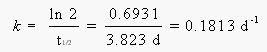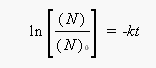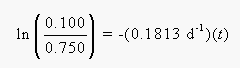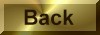Practice Problem 8

How long would it take for a sample of 222Rn that weighs 0.750 g to decay to 0.100 g?  Assume a half-life for 222Rn of 3.823 days.

Solution

We can start by calculating the rate constant for this decay from the half-life:We then turn to the integrated form of the first-order rate law:The ratio of the number of atoms that remain in the sample to the number of atoms present initailly is the same as the ratio of grams at the end of the time period to the number of grams present initially:Solving for t, we find that is takes 11.1 days for 0.750 g of  222Rn to decay to 0.100 g of this nuclide.